# Vectors Directed Line Segments and Geometric Vectors A

• Slides: 23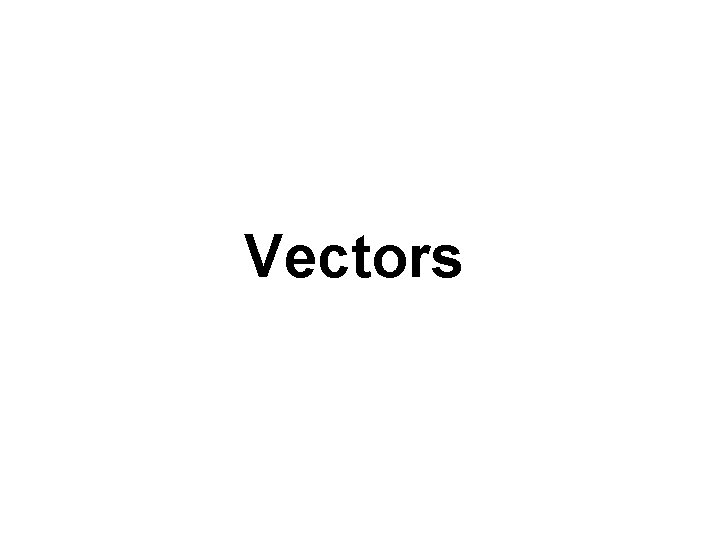VectorsDirected Line Segments and Geometric Vectors A line segment to which a direction has been assigned is called a directed line segment. The figure below shows a directed line segment form P to Q. We call P the initial point and Q the terminal point. We denote this directed line segment by PQ. Q Initial point Terminal point P The magnitude of the directed line segment PQ is its length. We denote this by || PQ ||. Thus, || PQ || is the distance from point P to point Q. Because distance is nonnegative, vectors do not have negative magnitudes. Geometrically, a vector is a directed line segment. Vectors are often denoted by a boldface letter, such as v. If a vector v has the same magnitude and the same direction as the directed line segment PQ, we write v = PQ.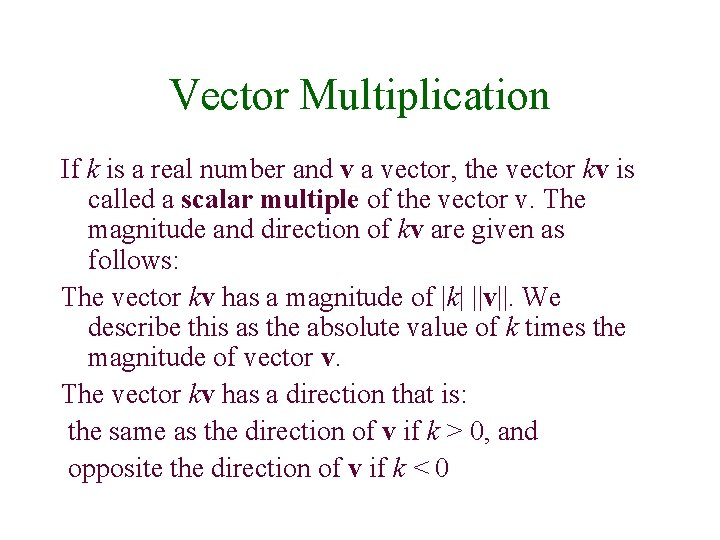Vector Multiplication If k is a real number and v a vector, the vector kv is called a scalar multiple of the vector v. The magnitude and direction of kv are given as follows: The vector kv has a magnitude of |k| ||v||. We describe this as the absolute value of k times the magnitude of vector v. The vector kv has a direction that is: the same as the direction of v if k > 0, and opposite the direction of v if k < 0The Geometric Method for Adding Two Vectors A geometric method for adding two vectors is shown below. The sum of u + v is called the resultant vector. Here is how we find this vector. 1. Position u and v so the terminal point of u extends from the initial point of v. 2. The resultant vector, u + v, extends from the initial point of u to the terminal point of v. Resultant vector u+v u Initial point of u v Terminal point of vThe Geometric Method for the Difference of Two Vectors The difference of two vectors, v – u, is defined as v – u = v + (-u), where –u is the scalar multiplication of u and – 1: -1 u. The difference v – u is shown below geometrically. -u v–u -u v u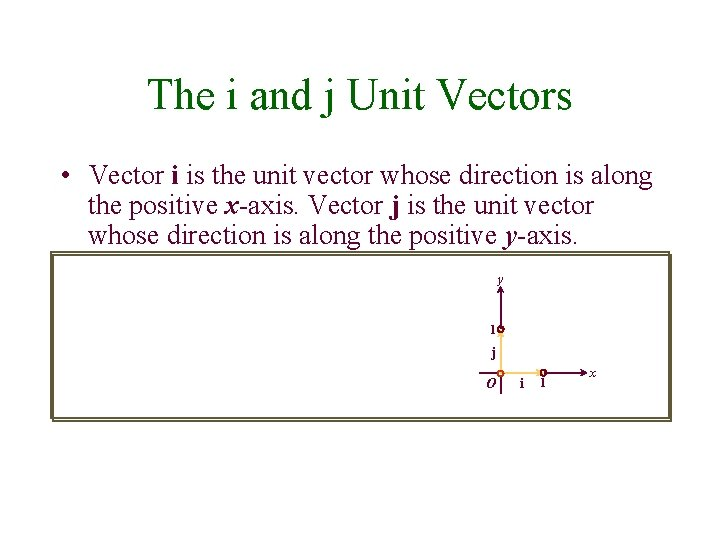The i and j Unit Vectors • Vector i is the unit vector whose direction is along the positive x-axis. Vector j is the unit vector whose direction is along the positive y-axis. y 1 j O i 1 x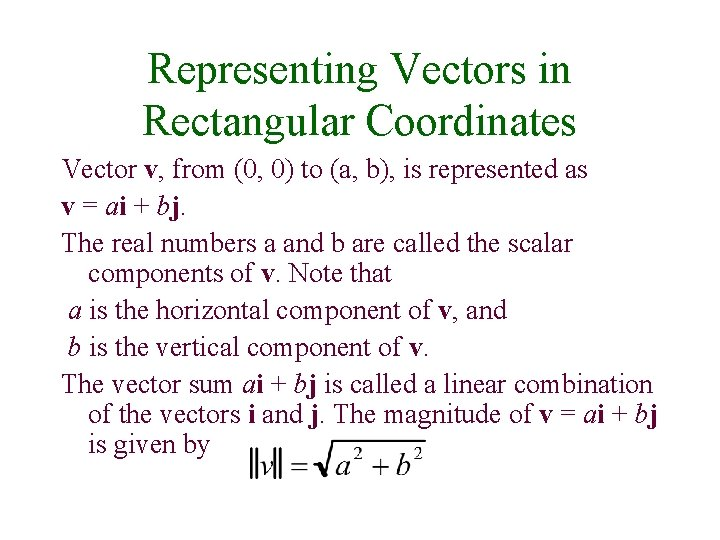Representing Vectors in Rectangular Coordinates Vector v, from (0, 0) to (a, b), is represented as v = ai + bj. The real numbers a and b are called the scalar components of v. Note that a is the horizontal component of v, and b is the vertical component of v. The vector sum ai + bj is called a linear combination of the vectors i and j. The magnitude of v = ai + bj is given by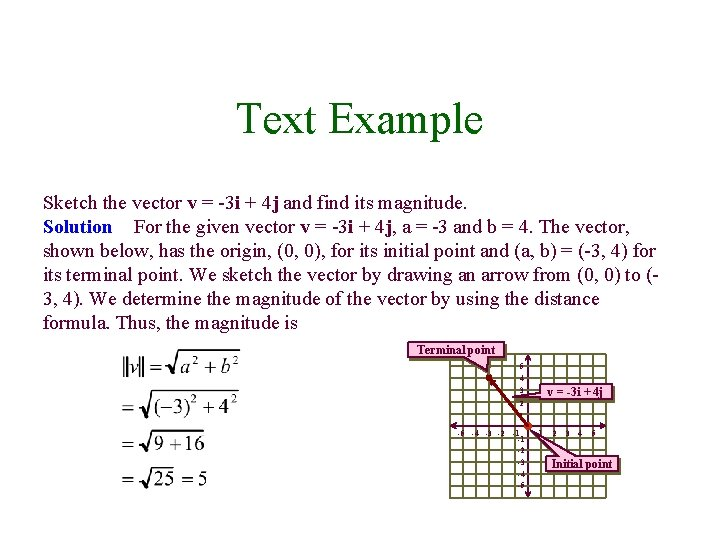Text Example Sketch the vector v = -3 i + 4 j and find its magnitude. Solution For the given vector v = -3 i + 4 j, a = -3 and b = 4. The vector, shown below, has the origin, (0, 0), for its initial point and (a, b) = (-3, 4) for its terminal point. We sketch the vector by drawing an arrow from (0, 0) to (3, 4). We determine the magnitude of the vector by using the distance formula. Thus, the magnitude is Terminal point 5 4 v = -3 i + 4 j 3 2 1 -5 -4 -3 -2 -1 -1 -2 -3 -4 -5 1 2 3 4 5 Initial point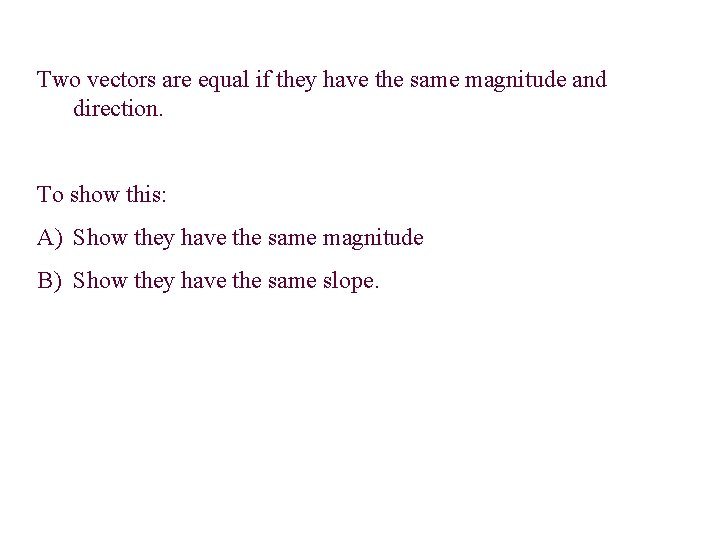Two vectors are equal if they have the same magnitude and direction. To show this: A) Show they have the same magnitude B) Show they have the same slope.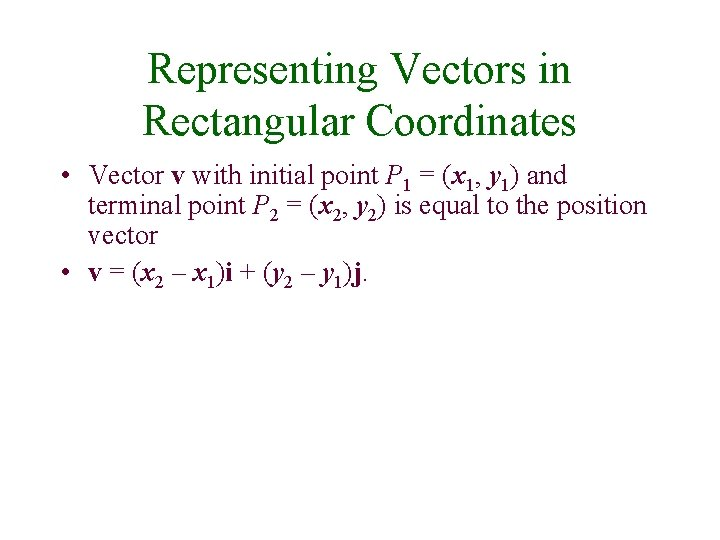Representing Vectors in Rectangular Coordinates • Vector v with initial point P 1 = (x 1, y 1) and terminal point P 2 = (x 2, y 2) is equal to the position vector • v = (x 2 – x 1)i + (y 2 – y 1)j.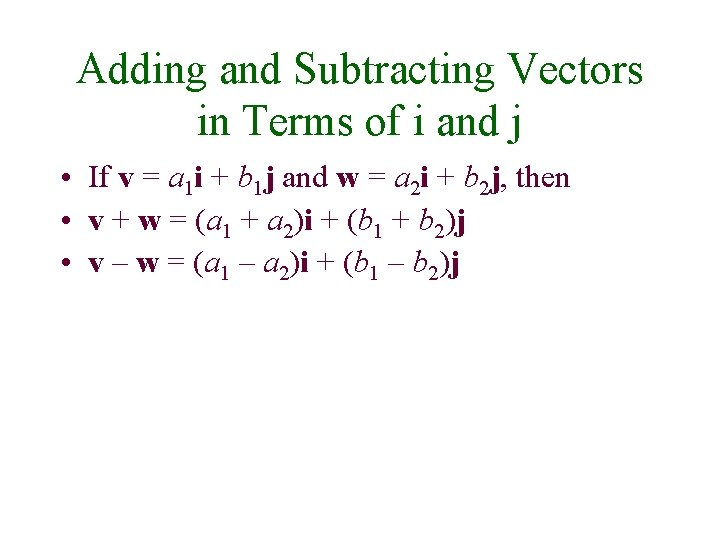Adding and Subtracting Vectors in Terms of i and j • If v = a 1 i + b 1 j and w = a 2 i + b 2 j, then • v + w = (a 1 + a 2)i + (b 1 + b 2)j • v – w = (a 1 – a 2)i + (b 1 – b 2)jText Example If v = 5 i + 4 j and w = 6 i – 9 j, find: a. v + w b. v – w. Solution • v + w = (5 i + 4 j) + (6 i – 9 j) = (5 + 6)i + [4 + (-9)]j vertical components. = 11 i – 5 j • v + w = (5 i + 4 j) – (6 i – 9 j) = (5 – 6)i + [4 – (-9)]j the vertical components. = -i + 13 j These are the given vectors. Add the horizontal components. Add the Simplify. These are the given vectors. Subtract the horizontal components. Subtract Simplify.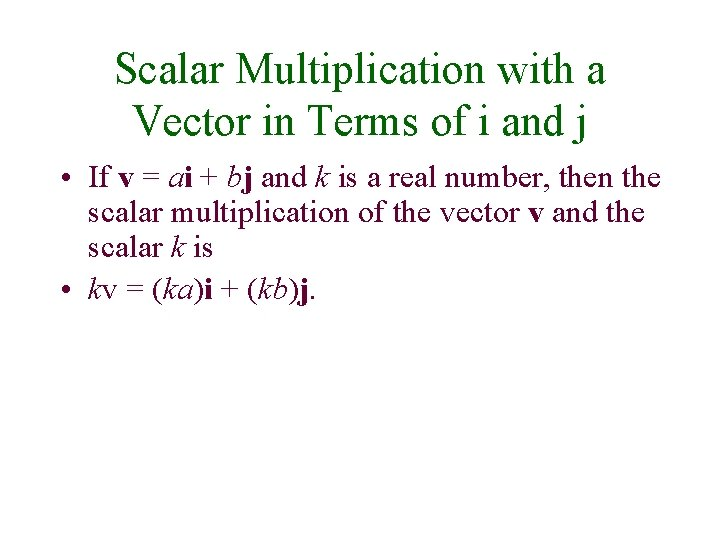Scalar Multiplication with a Vector in Terms of i and j • If v = ai + bj and k is a real number, then the scalar multiplication of the vector v and the scalar k is • kv = (ka)i + (kb)j.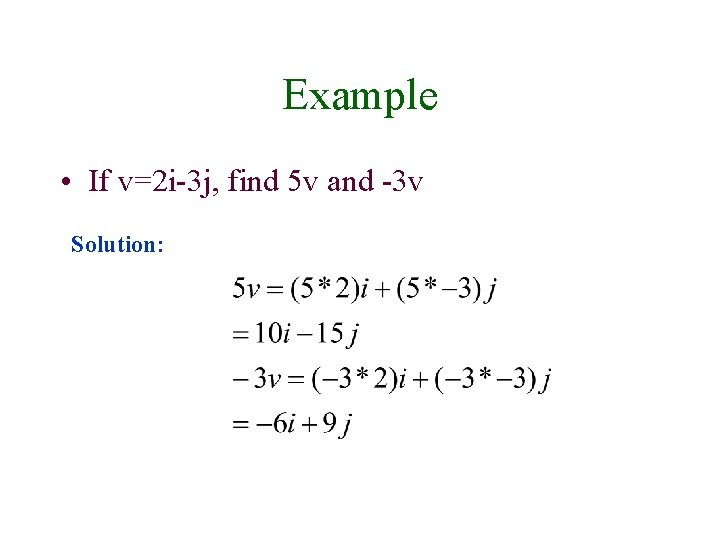Example • If v=2 i-3 j, find 5 v and -3 v Solution:The Zero Vector • The vector whose magnitude is 0 is called the zero vector, 0. The zero vector is assigned no direction. It can be expressed in terms of i and j using • 0 = 0 i + 0 j.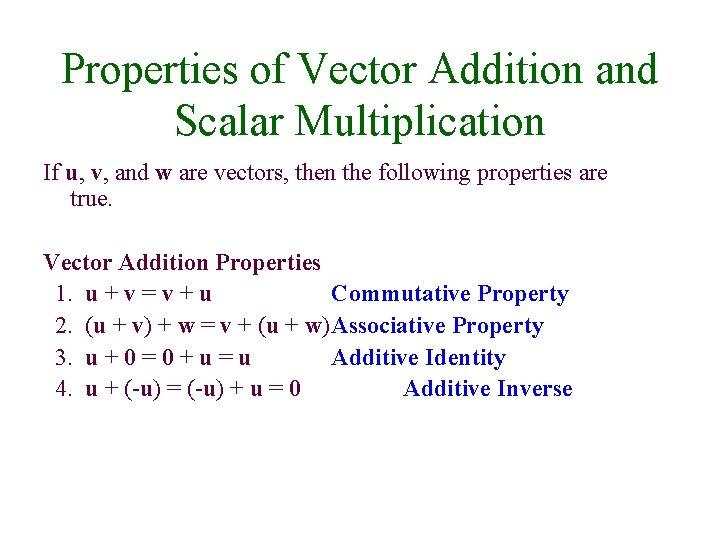Properties of Vector Addition and Scalar Multiplication If u, v, and w are vectors, then the following properties are true. Vector Addition Properties 1. u + v = v + u Commutative Property 2. (u + v) + w = v + (u + w)Associative Property 3. u + 0 = 0 + u = u Additive Identity 4. u + (-u) = (-u) + u = 0 Additive Inverse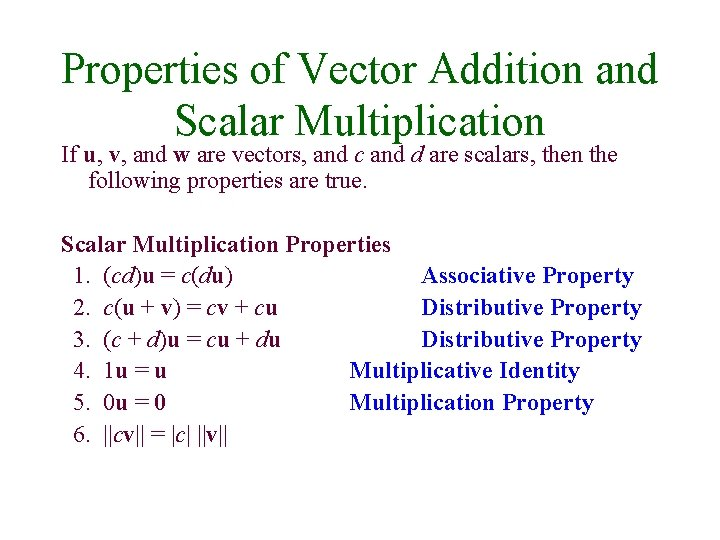Properties of Vector Addition and Scalar Multiplication If u, v, and w are vectors, and c and d are scalars, then the following properties are true. Scalar Multiplication Properties 1. (cd)u = c(du) Associative Property 2. c(u + v) = cv + cu Distributive Property 3. (c + d)u = cu + du Distributive Property 4. 1 u = u Multiplicative Identity 5. 0 u = 0 Multiplication Property 6. ||cv|| = |c| ||v||Finding the Unit Vector that Has the Same Direction as a Given Nonzero Vector v • For any nonzero vector v, the vector • is a unit vector that has the same direction as v. To find this vector, divide v by its magnitude.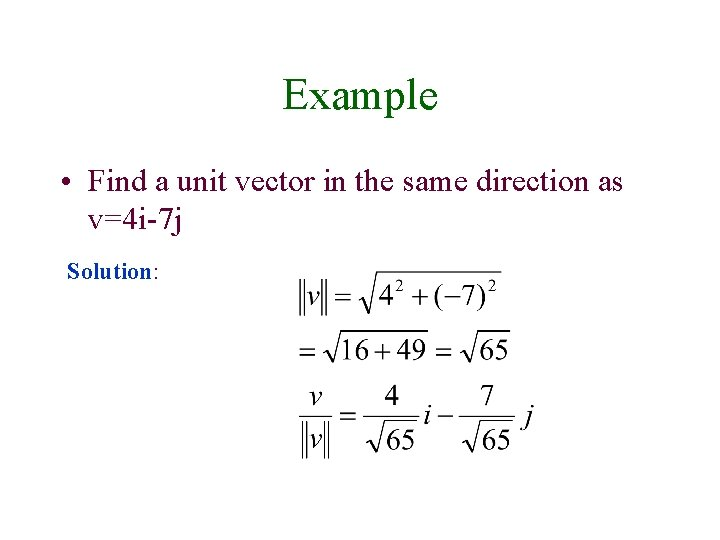Example • Find a unit vector in the same direction as v=4 i-7 j Solution: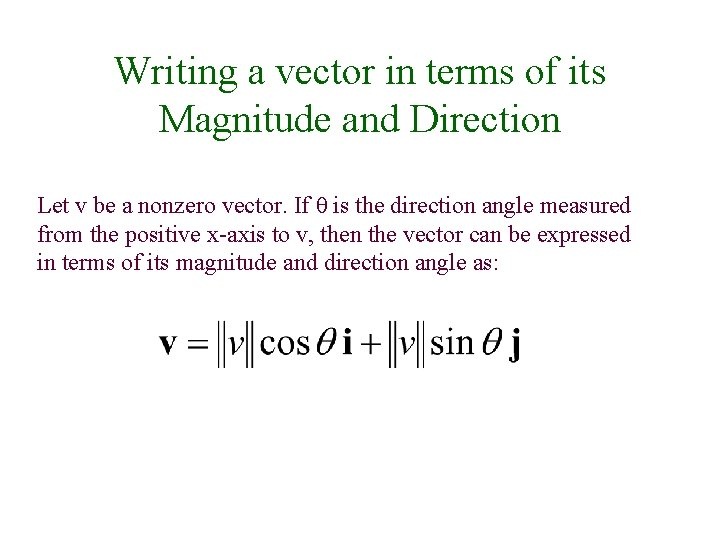Writing a vector in terms of its Magnitude and Direction Let v be a nonzero vector. If θ is the direction angle measured from the positive x-axis to v, then the vector can be expressed in terms of its magnitude and direction angle as: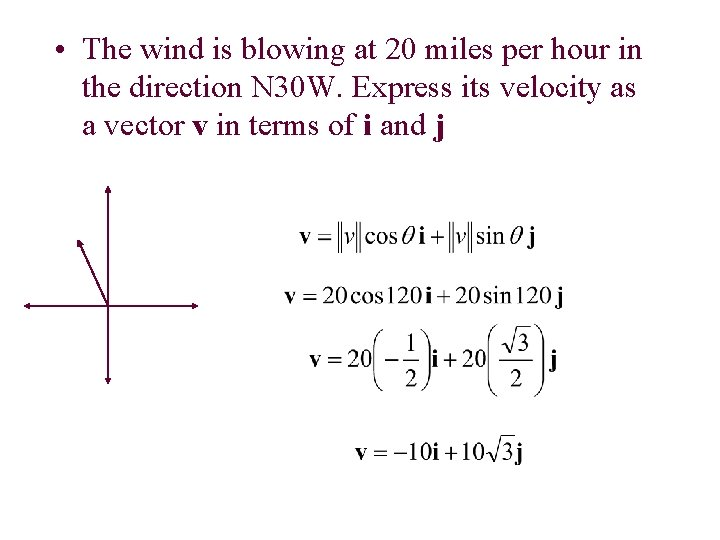• The wind is blowing at 20 miles per hour in the direction N 30 W. Express its velocity as a vector v in terms of i and jApplications (Resultant Force) • A vector that represents a pull or push of some type is called a force vector. Resulatant Force = F 1 + F 2 If resultant force is zero vector, then the object is not moving!Two forces F 1 and F 2 of magnitude 10 and 30 pounds respectively, act on an object. The direction of F 1 is N 20 E and the direction of F 2 is N 65 E. Find the magnitude and the direction of the resultant force. The two given forces are equivalent to a single force of approximately 37. 74 pounds with a direction angle of 35. 8 degrees.